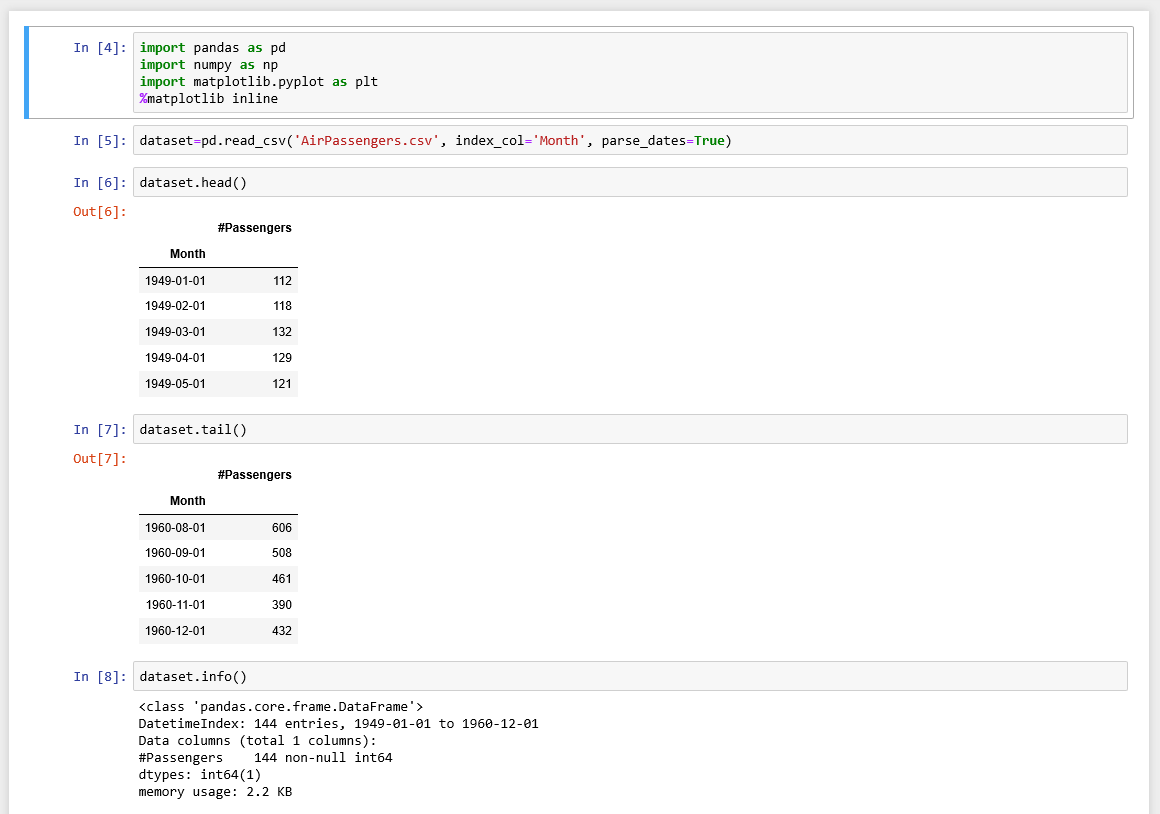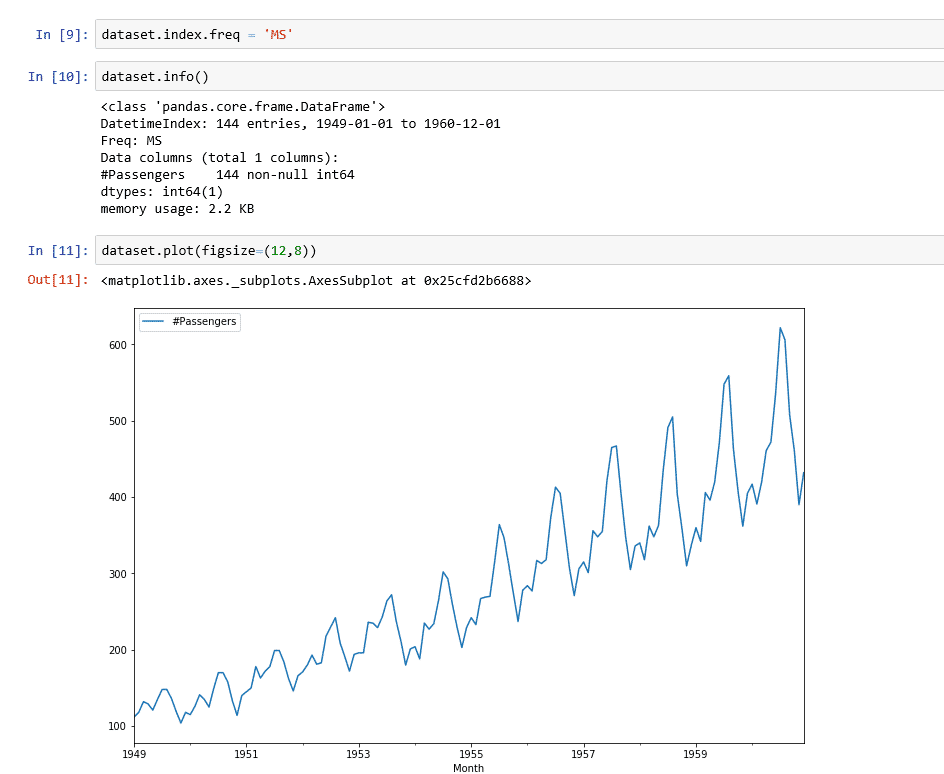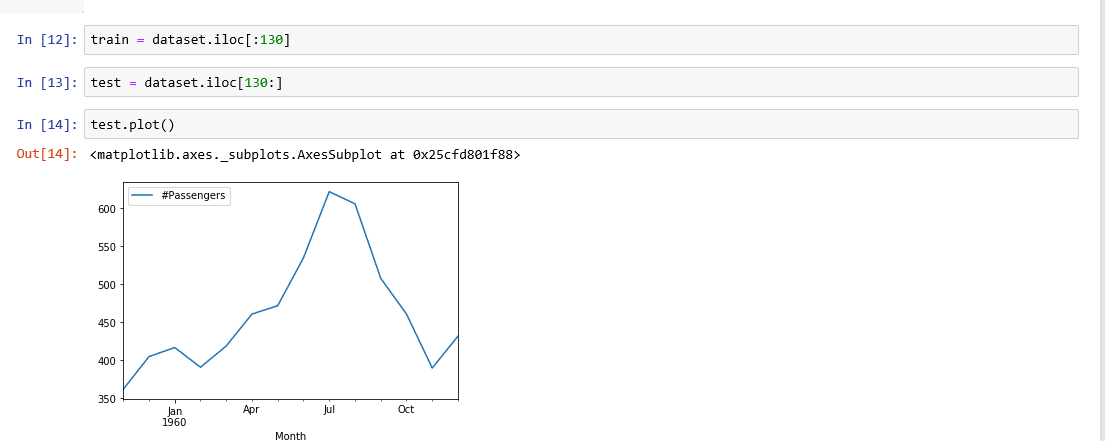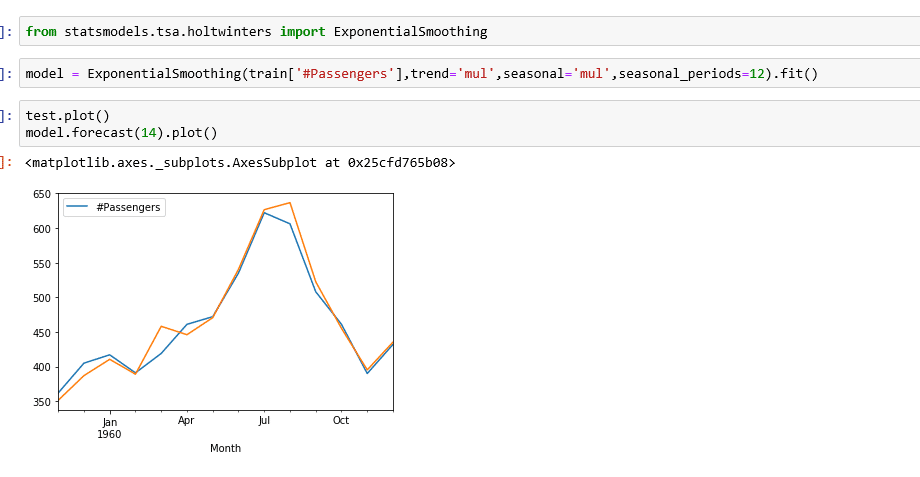# Forecasting with Python and Power BI

We are using an airline passenger dataset that you can get from Kaggle, which shows us the data from 1949 to 1960 for airline passengers.  Please find the PBIX file on my Github

Load in my dependencies, which are pandas and numpy, and Matplotlib. I’m just going to import those in. And then once I have that, I’m going to read in the CSV that has our airline passenger data. While I’m reading that in, by bringing that file name, I’m going to set the index column as the date column, which is called month in this data set. I’m going to ensure that we can parse the dates.

Watch the VideoIf I check the head of that data set, you can see that the head has the month as the index, and then the number of passengers as a row.  The data starts at 1949 and then the tail is from 1960. If I look at the info, we also can see that same data as 144 months. You can see it spans from 1949 to 1960.

## Triple Exponential Smoothing Forecast

The model that we’re going to be using is triple exponential smoothing the Holt-Winters triple exponential smoothing model for our forecast. We’re going to get that model from statsmodel.

from statsmodel.tsa.holtwinters import ExponentialSmoothing

We’re going to use that as a function, but we need to set the parameters as that function and fit our data to that model.

Remember, our data looks like it’s growing exponentially instead of in a linear fashion. So I’m going to use the multiplicative model instead of the additive model for defining our data. If you look at these two images here, you can see the additive seasonality is more linear and then multiplicative is a little bit more on the exponential side of things.### Create a training and test set### Load in your machine learning model

So once I’ve defined that model, Fit it to our training data using the fit function. Plot the test data and plot the forecast using the forecast function to forecast 14 months forward from my training data,

If we plot both of those, we can see that the model adheres quite well to our data. Now that we know that we have a decent model, we can also adjust how many periods forward we are forecasting using that forecast function.So once we have this, we can create a dashboard that does the same thing but let’s look at how we use that code in Power BI.  Access the Python scripting with Transform Data. Next and go over to run Python. The same steps from Jupyter Notebook are required. You can see the code below somewhat truncated, Simply copy and paste it in.

```import pandas as pd
import numpy as np
dataset.set_index('Month')
dataset.index.freq='MS'
from statsmodels.tsa.holtwinters import ExponentialSmoothing
model = ExponentialSmoothing(dataset['#Passengers'],trend='mul',seasonal='mul',seasonal_periods=12).fit()
range = pd.date_range('01-01-1961',periods=36,freq='MS')
predictions = model.forecast(36)predictions_range = pd.DataFrame({'#Passengers':predictions,'Month':range})```#### Gaelim Holland

Subscribe
Notify ofInline FeedbacksAjay
2 years ago
• Great Solution using Python, could you please pbix file.Andrei
1 year ago

Great presentation.
If I get it right you are doing the model training right in the PBI Transform Data step.
I assume for some complex models and large training sets it is better to train the model outside of PBI, persist it into a file and in the PBI Transform just load the model and calculate the predictions.

Is it possible to do this in an automated way?

Thanks!Ramzy
6 months ago

do you have a link to the jupyter notebook? Id like to use this on my PowerBI file.. Thank you!